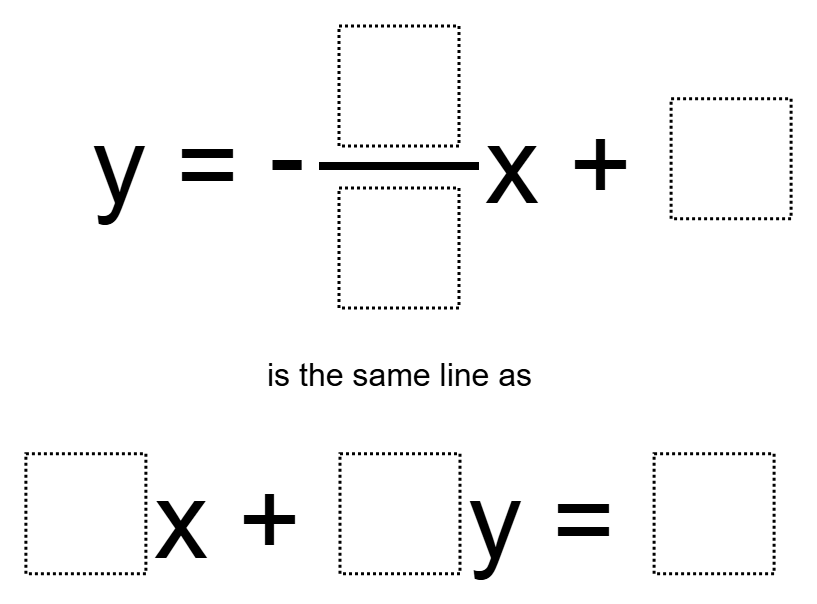Home > Grade 8 > Functions > Equivalent lines in slope-intercept and standard form

# Equivalent lines in slope-intercept and standard form

Directions: Using the whole numbers 1 through 9, no more than one time each, complete the statement below.### Hint

Which numbers are related in the two equations? How can we use this information to fill in the numbers that are “related”?

y=-3/1x+4 and 6x+2y=8

Source: Andy Schwen

## Square Root Expression

Directions: Use the digits 1 to 9, at most one time each, to fill in …

### One comment

1.Students also found:

-9/3x+4 6x+2y=8
-4/1x+3 8x+2y=6